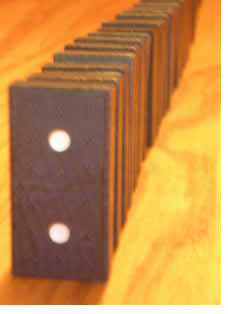# An Introduction to Mathematical Induction

##### Age 16 to 18

Published 2011 Revised 2018

Quite often in mathematics we find ourselves wanting to prove a statement that we think is true for every natural number $n$.

For example, you may have met the formula $\frac{1}{6}n(n+1)(2n+1)$ for the sum $$\sum_{i=1}^n i^2=1^2+2^2+\ldots+n^2.$$ We can try some values of $n$, and see that the formula seems to be right: \begin{eqnarray} n=1: & & \sum_{i=1}^n i^2 = 1^2 = 1;\\ & & \frac{1}{6}n(n+1)(2n+1) = \frac{1}{6}\times 1\times 2\times 3 = 1\\ \\ n=2: & & \sum_{i=1}^n i^2 = 1^2 + 2^2 = 1+4 = 5;\\ & & \frac{1}{6}n(n+1)(2n+1) = \frac{1}{6}\times 2\times 3\times 5 = 5\\ \\ n=3: & & \sum_{i=1}^n i^2 = 1^2 + 2^2 + 3^2 = 1+4+9 = 14;\\ & & \frac{1}{6}n(n+1)(2n+1) = \frac{1}{6}\times 3\times 4\times 7 = 14.\\ \end{eqnarray} But we want to prove that this is true for all positive integers $n$, and it's going to be impossible if we try to do this by putting in all possible values! Instead, we're going to have to be a bit more cunning$\ldots$Have you ever played that game with dominoes where you line them up on end and then, by knocking over the first one, knock over the whole lot? You can think of proof by induction as the mathematical equivalent (although it does involve infinitely many dominoes!). Suppose that we have a statement $P(n)$, and that we want to show that it's true for all $n$. So in our example above, $P(n)$ is: $$\sum_{i=1}^n i^2 = \frac{1}{6}n(n+1) (2n+1)$$ Think of each $P(n)$ as a domino. If we can show that $P(1)$ is true (that is, we can knock over the first domino)and that if $P(n)$ is true then so is $P(n+1)$ (knocking over one domino means the next one will also fall over), do you agree that we've then shown that $P(n)$ is true for all $n\geq 1$ (because all of the dominoes will fall over)?
 We'll know that $P(1)$ is true, so we'll know that $P(1+1)=P(2)$ is true, so we'll know that $P(2+1)=P(3)$ is true, so we'll know that $P(3+1)=P(4)$ is true, $\ldots$ You get the idea.Let's go back to our example from above, about sums of squares, and use induction to prove the result. We know that $P(1)$ is true (we did that before!). Now let's see what would happen if we knew that $P(n)$ is true. Then we'd know that $\sum_{i=1}^n i^2 = \frac{1}{6}n(n+1)(2n+1)$. So what can we say about $\sum_{i=1}^{n+1} i^2$? Well, \begin{eqnarray} \sum_{i=1}^{n+1} i^2 & = & (n+1)^2 + \sum_{i=1}^n i^2\\ & = & (n+1)^2 + \frac{1}{6}n(n+1)(2n+1)\quad\textrm{(by the inductive hypothesis)}\\ & = & \frac{1}{6}(n+1)\left[6(n+1)+n(2n+1)\right]\\ & = & \frac{1}{6}(n+1)\left[6n+6+2n^2+n\right]\\ & = & \frac{1}{6}(n+1)\left[2n^2+7n+6\right]\\ & = & \frac{1}{6}(n+1)(n+2)(2n+3). \end{eqnarray} This looks familiar; what is $P(n+1)$? Well, let's substitute $n+1$ in place of $n$ in our statement of $P(n)$. So we're aiming for $$\sum_{i=1}^{n+1} i^2 = \frac{1}{6}(n+1)(n+2)(2(n+1)+1)=\frac{1}{6}(n+1)(n+2)(2n+3),$$ which is exactly what we've just got! (Working out what you're aiming for can often give you an idea of how to manipulate the algebra.) So we've shown that if $P(n)$ is true, then $P(n+1)$ is true. Since we also know that $P(1)$ is true, we know that $P(2)$ is true, so $P(3)$ is true, so $P(4)$ is true, so $\ldots$ In other words, we've shown that $P(n)$ is true for all $n\geq 1$, by mathematical induction.

Warning: When encountering induction for the first time, people usually remember to show "if $P(n)$ is true then $P(n+1)$ is true'', because that's what induction is really about, but a common mistake is to forget to show that $P(1)$ is true. Proof by induction is a two-stage process, even if one stage is usually very easy. The dominoes won't fall over unless you knock over the first one!

Don't forget that your first domino doesn't have to be $P(1)$. It could be $P(2)$, or $P(19)$, or $P(1000000)$. For example, we can use induction to show $3^n> n^3$ for $n\geq 4$ (see the exercises below)

One last thing: induction is only a method of proof . For example, if you're trying to sum a list of numbers and have a guess for the answer, then you may be able to use induction to prove it. But you can't use induction to find the answer in the first place. Also, there are often other methods of proof: I've given some examples below of things that you might like to try to prove by induction, but several of them can be proved at least as easily by other methods (indeed, you've probably seen some proved by other methods).

1. Prove by induction that $$\sum_{i=1}^n i^3 = \frac{1}{4}n^2(n+1)^2\textrm{ for }n\geq 1.$$
2. Use induction to show that $$\sum_{i=1}^n r^{i-1}=\frac{r^n - 1}{r-1}\textrm{ for }n\geq 1\textrm{ and }r\neq 1.$$
3. Use induction to show that $3^n> n^3$ for $n\geq 4$. (Note that you have to start at $n=4$ as the result isn't true for $n=3$!)
4. Use induction to show that $$\left(\begin{array}{c}n+1\\r\end{array}\right) = \left(\begin{array}{c}n\\r\end{array}\right) + \left(\begin{array}{c}n\\r-1\end{array}\right)\textrm{ for }n\geq 1$$ (where $\left(\begin{array}{c}n\\r\end{array}\right)$ is the binomial coefficient). (You will need to use induction on $n$ and keep $r$ fixed). Remark: This is rather fiddly; starting with the right-hand side will make things easier. This example is an excellent lesson in why you should not always use induction: thinking about counting things will help you prove the result much more easily. Can you see how?
5. Use induction to show that $4^n+6n$ is divisible by 6 for $n\geq 1$. [No this is not a misprint. What part of the argument works? What part fails?]
6. Use induction to show that $4^n + 6n -1$ is divisible by 9 for $n\geq 1$.